# Construction-specific Math

## Estimating Math Concepts

The vast majority of construction estimating problems can be solved using basic addition, subtraction, multiplication, and division skills coupled with a knowledge of simple geometry functions, such as length, perimeter, area, and volume. Additionally, an understanding of a few simple trigonometric functions will be used. This chapter will review these basic concepts and mastering these concepts will enable you to perform most estimating calculations. The review will begin with the concept of length.

### Length

The ability to add, subtract, multiply and divide length is the most fundamental estimating mathematical skill. It is used constantly when performing quantity takeoffs and estimates. The biggest challenge faced with length calculations is that dimensions on construction plans and drawings prepared using the English system of measurement are given in feet, inches, and fractions of an inch. It is common to see length measurements listed in the following format:

11′- 10 ¼″

This measurement would be read as eleven feet, ten and one quarter-of-an-inch. This is a convenient form of measurement for the trade craftsman in the field to use as it corresponds to the layout of most rulers and tape measures in the English system. It is, however, problematic for the construction estimator because he or she frequently needs to add, subtract, multiply, or divide by these fractional numbers. This problem is not as prevalent in the metric system. In the metric system, it is easy to convert 1.19 meters into 119 centimeters. Due to the straightforward nature of the metric system, complicated conversions are generally unnecessary. This chapter will primarily deal with the English system.

Figure 2-1 shows an example of rectangular shaped concrete footing for a small shed.

The dimensions for the footing are given to the center of the footing, and the estimator would need to calculate the total length of the footings by adding up the dimensions of each of the sides.

The manual calculation would be as follows:

15′- 4″

11′- 10 ¼″

15′- 4″

+ 11′- 10 ¼″

To solve this equation, the estimator would first need to add the fractional inches.

¼″ + ¼″ = ½″

If the total of the fractions is greater than 1 inch, then the result would need to be converted to inches and fractions. Because the example is less than 1 inch, the 1/2 inch can be left in the fractional form. Next, the inches would be added together, and if the total is greater than 12 inches, it would need to be converted into feet and inches by dividing the total by 12.

4″ + 10″ + 4″ + 10″ = 28″ ÷ 12″ = 2′- 4″

The 2 feet from the inch column would be carried over to the feet column, and the total feet would be added together.

2′ + 15′ + 11′ + 15′ + 11′ = 54′

54′ - 4 ½″

Calculations like this are very accurate, but it can be time-consuming to perform. The estimator should not only strive for accuracy but also speed. One method of accelerating the process is to convert the fractional numbers into a decimal format. Modern calculators can do this quite easily. In addition, formulas can be written in Microsoft Excel to speed up the process; however, the estimator should strive to do basic fraction-to-decimal conversions in his or her head.

Fractions of an inch are converted to decimal form by dividing the numerator by the denominator. The one-quarter-inch fraction in the previous example can be converted to a decimal by dividing the 1 by the 4, as shown below.

1 ÷ 4 = 0.25

Any fraction could be converted to decimal form using this method, but accuracy closer than one-quarter-of-an-inch is not necessary. Memorizing the following three fractional conversions will be sufficient in most construction estimating circumstances:

¼″ = 0.25″

½″ = 0.5″

¾″ = 0.75″

Converting inches to feet is done in similar fashion, except that the inch dimension is divided by 12. The following chart shows the inch conversions:

1″ ÷ 12″ = 0.083′

2″ ÷ 12″ = 0.167′

3″ ÷ 12″ = 0.250′

4″ ÷ 12″ = 0.333′

5″ ÷ 12″ = 0.417’

6″ ÷ 12″ = 0.500′

7″ ÷ 12″ = 0.583′

8″ ÷ 12″ = 0.667′

9″ ÷ 12″ = 0.750′

10″ ÷ 12″ = 0.833′

11″ ÷ 12″ = 0.917′

As is the case with fractions, memorizing a few of the inch conversions will be sufficient in most cases. The five conversions highlighted above should be memorized.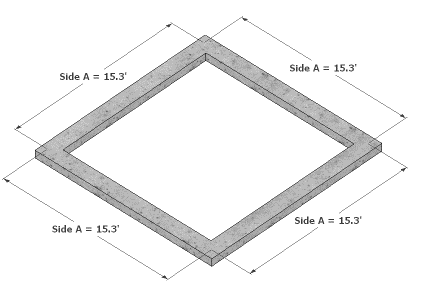Figure 2-2 Perimeter of a square footing where each side is 15.3 feet’ long

In practical reality, the estimator should be able to quickly perform a rough calculation for the length of the shed footings in his head by converting the fractional dimensions to decimal form and rounding close dimensions. Using this method, the estimator would convert the 15′ 4″ length to 15.3′ and the 11′ 10 ¼″ would be rounded up to 12′ leaving a simple calculation of

12.0' + 15.3' + 12.0' + 15.3' = 54.6'

In this example, the 54.6′ length would be close enough to get an accurate estimate of the length of the footings and would result in about 1/5 of a cubic foot (one or two shovels full) of additional concrete for the footing.

### Perimeter

The previous example with the concrete footings shows an important use of length calculations in determining the perimeter of various shapes. Calculating the perimeter of basic square or rectangular shapes could be done by adding up the length of each side, as was shown in the previous example; however, slightly more complex formulas would be more useful.

#### Perimeter of Squares and Rectangles

A square is a rectangle shape where all the sides are the same length. The perimeter of a square where the length of each side is 15.3′ long, as shown in Figure 2-2, could be written as

15.3′ + 15.3′ + 15.3′ + 15.3′ = 61.2′

This would work, but as will be shown when creating formulas for the spreadsheet calculator, it can get unwieldy to use. A simpler formula would be to multiply the length of the single side by 4.

Length of Side A × 4

15.3′ × 4 = 61.2′

The formula for a rectangle would be slightly more complex because it involves sides of two different lengths. Instead of using the previous example for the rectangular footing in Figure 2-3, the formula would be written as the Length of Side A × 2 + Length of Side B × 2.

15.3′ × 2 + 12.0′ × 2 = 54.6′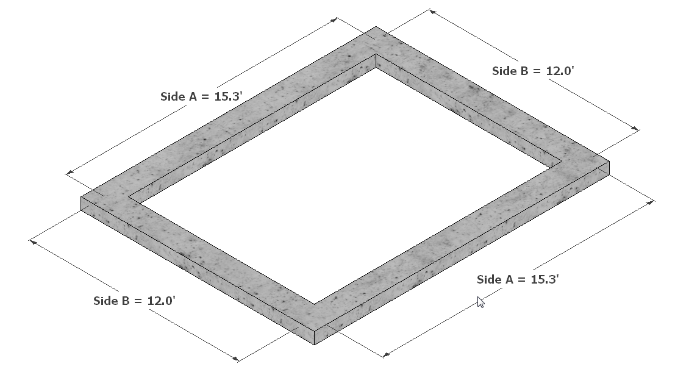Figure 2-3 Perimeter of a rectangle footing with two side being 15.3 feet and two sides being 12.0 feet

#### Perimeter of Circles

The traditional formula for calculating the perimeter of a circle is

Radius is the distance from the center of a circle to its edge, and ∏ is the symbol for the Greek letter pi (pronounced pie) and represents the mathematical constant of 3.14159…, which is a ratio of a circle’s diameter to its circumference. The diameter of a circle is the distance across the circle at its center point. Figure 2-4 shows a circle whose radius is 10’ and corresponding diameter is 20′.

The formulas for calculating the perimeter of the circle in Figure 2-4 would then be

10′ × 2 × 3.14159 = 62.83′

Or

Diameter × ∏

20′ × 3.14159 = 62.83′

Both formulas will result in the correct answer.

##### Perimeter of Partial Circles

The estimator may need to calculate the perimeter distance of a partial circle, or what is called the arc length of the partial circle. A common way to do this is to take into account the angle of degree that the partial circle contains. A full circle is divided into 360 degrees. A partial circle would represent some fraction of the full circle. Figure 2-5 shows the same 20-foot diameter circle that is used in Figure 2-4. The full circle is 360°, and the partial circle arc length is 90°.

The arc length of the partial circle would be calculated by first determining the fractional part of the circle the arc represents. In this case, the 90° of the arc would be divided by the 360° of the full circle and the results reduced to a decimal equivalent.

$$\frac{90^{\circ}}{360^{\circ}}\:=\:\frac{1}{4}$$
$$\frac{1}{4}\:=\:0.25$$

The length of the arc would then be calculated by the following formula:

Diameter × ∏ × 0.25

20′ × 3.14159 × 0.25 = 15.71′

The arc length of other partial circles can be calculated in a similar fashion.

#### Perimeter of Triangles

Calculating the perimeter of a triangle depends upon the type of triangle. The three most common triangle shapes encountered in construction estimating are right triangles, equilateral triangles, and isometric triangles. All triangles are composed of three angles with the total of all three angles equaling 180 degrees. The difference in each type of triangle is how the angles come together to form the shape. Each triangle requires a slightly different method for determining the perimeter.

##### Perimeter of Right Triangles

Right triangles are triangles that have one 90° angle. The total of the other two angles equal 90°. Figure 2-11 shows several examples of right triangles: an isosceles triangle, a 30-60-90 triangle, and a general triangle. An isosceles triangle has two legs of the same length and two 45° angles. A 30-60-90 triangle has one 30° angle, one 60° angle, and one 90° angle. The two non-90° angles of a general triangle are a combination of angles other than 30°, 45°, and 60°. Each type of right triangle has some common elements: two legs, usually identified as Side A and Side B. The intersection of Sides A and B form the 90° angle. The side opposite the 90° angle is the hypotenuse.

The length of the hypotenuse is determined by using the Pythagorean Theorem, which states that the square of the hypotenuse is equal to the square of the sum of the two sides. This is commonly referred to as

A2 + B2 = C2

Using the Pythagorean Theorem, the hypotenuse for the 30-60-90 triangle in Figure 2-11 could be solved by following the equation Side A2 + Side B2 = Side C2.

11 ft. − 6 ½ in.2 + 20 ft.2 = Side C2

11.54 ft.2 + 20 ft.2 = C2

133.17 + 400 = C2

$$\sqrt{533.17\:ft.}\:=\:23.09\:ft.$$

The perimeter of the same triangle would then be solved by following the equation Length Side A + Length Side B + Length Side C.

11.54′ + 20′ + 23.09′ = 54.63′

A calculator would commonly be used to determine the square root of 533.17, but it also could be used to solve the entire 30-60-90 right triangle problem. Calculators are programmed to use mathematical order of operations when solving equations; an understanding of this concept is essential to solve construction estimating problems and writing formulas in Microsoft Excel.

##### Math Order of Operations

Most students are familiar with the phrase “Please Excuse My Dear Aunt Sally,” which is used to help remember the acronym PEMDAS, which represents the order in which mathematical operations in an equation are solved. The mathematical order is

1. Parenthesis
2. Exponents
3. Multiplication
4. Division
6. Subtraction

In the previous 30-60-90 triangle example, the first step to solving the equation would be to convert the 11′6 ½″ to decimal form. The decimal equivalent of the ½″ fraction is 0.5″ (this fractional conversion should have been memorized), and when it is added to the 6-inch length, it equals 6.5 inches. Next, 6.5 inches will be converted to a decimal foot equivalent by dividing by 12 and adding the decimal inches to the 11 feet. Mathematically the equation would be

11 + 6.5 ÷ 12 = 11.54167

This equation works because the order of operations requires division before the addition, and the equation would be solved in the following order

Step 1: 6.5 ÷ 12 = 0.54167

Step 2: 0.54167 + 11 = 11.54167

##### Perimeter of Equilateral Triangles

Determining the perimeter of equilateral triangles is a little simpler because all three legs of an equilateral triangle are the same length, and each of the angles are the same at 60°. Figure 2-15 shows an equilateral triangle with a length on each side of 20 feet.

Calculating the perimeter of this triangle is as simple as adding up the three sides, as shown below.

Side A + Side B + Side C

20′ + 20′ + 20′ = 60′

##### Calculating the Perimeter of Isometric Triangles

Isometric triangles have two sides that are the same length with two corresponding angles that are also the same. The third side is a different length, and the angle opposite the third side is not equal to either 45 or 60 degrees. Figure 2-16 shows an example of an isometric triangle in which the base is 20 feet long.

In the example in Figure 2-16, the base is 20 feet long, and the two similar angles are 26.6°. Calculating the perimeter of this shape is a little more complex. The simplest method involves separating the isometric triangle into two similar right triangles and then using trigonometric functions to solve for the length of Side B or Side C. Figure 2-17 shows the previous isometric triangle broken into two similar right triangles. The length of the base of each triangle is 1/2 of the previous length of 20 feet or 10 feet.

##### Using Cosine to Solve for Hypotenuse Length

The length of either Side B or Side C can be determined by using one of several methods using simple trigonometric functions. The first function that could be used is the Cosine function. The formula for doing this would be written as

$$\frac{1}{\cos26.6^{\circ}}\:\times\:10'$$

The simplest method of determining the Cosine of 26.6° is to use the Cosine function of a calculator. This could be done on most standard handheld scientific calculators. To determine the Cosine of 26.6°, simply type the angle degrees of 26.6 into the calculator and press the Cos key. This will display the cosine value of 26.6°, which is .8941. Next, the results will be divided into one. To do this, press the reciprocal key on the calculator (1/x). This will display 1.18375. Finally, multiply this number by half of the length of Side A (half of Side A of the isometric triangle), which is 10 feet. The results will be 11.18375 feet, which is the length of both Side B and Side C of the isometric triangle (Figure 2-18).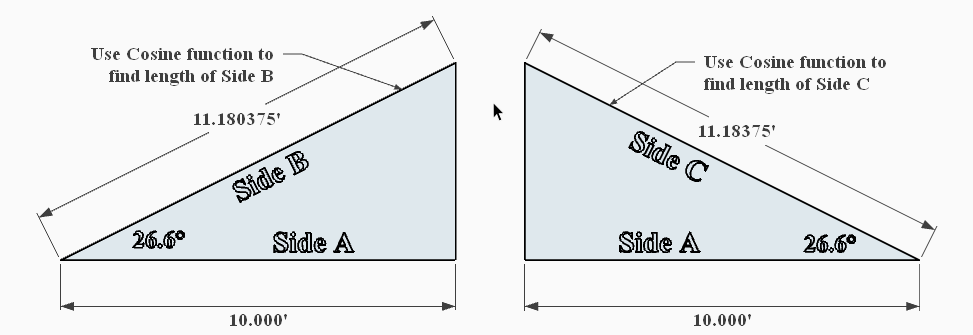Figure 2-18 Using Cosine to find length of Sides B and C

The perimeter of this isometric triangle would be the sum of the three sides.

20′ + 11.18375′ + 11.18375′ = 42.36750′

##### Using Tangent to Solve for Height

Another trigonometric function that could be used to solve the isometric triangle would be the tangent function. The tangent function can be used to solve the length of the side opposite the angle of a right triangle. The formula for doing this would be

$$\tan26.6^{\circ}\:\times\:10'$$

To determine the tangent of 26.6° using a calculator, enter 26.6 into the calculator and press the “tan” key. The calculator will return the tangent value of 0.500. Next, multiply this by the length of Side A of the triangle or 10 feet. The result will be 5 feet, which is the vertical height of the isometric triangle (Figure 2-20).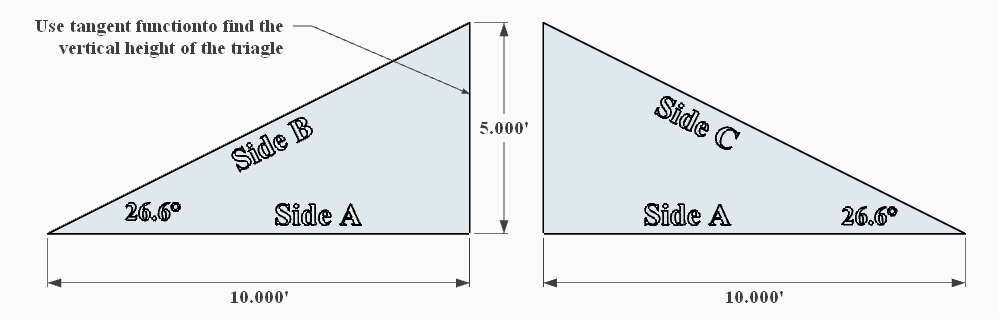Figure 2-20 Use tangent function to find vertical height of the isometric triangle.

With the vertical height of the isometric triangle known, the Pythagorean Theorem can be used to solve for the length of the hypotenuse.

##### Using Slope to Find the Perimeter of a Triangle

Construction documents often use the term slope to identify the angles of a right triangle. The slope is a ratio of the vertical height of the triangle to the horizontal length of the triangle. The vertical length is usually called the rise and horizontal length is called the run. The slope is commonly identified in the documents using a slope symbol, which is shown as a triangle where the run is 12 inches and the rise a portion of that. Figure 2-21 shows an example of a shed roof. The roof slope is indicated by slope symbol with the run shown as 12 inches and the rise of 6 inches. This may be expressed as either 6:12 or 6/12.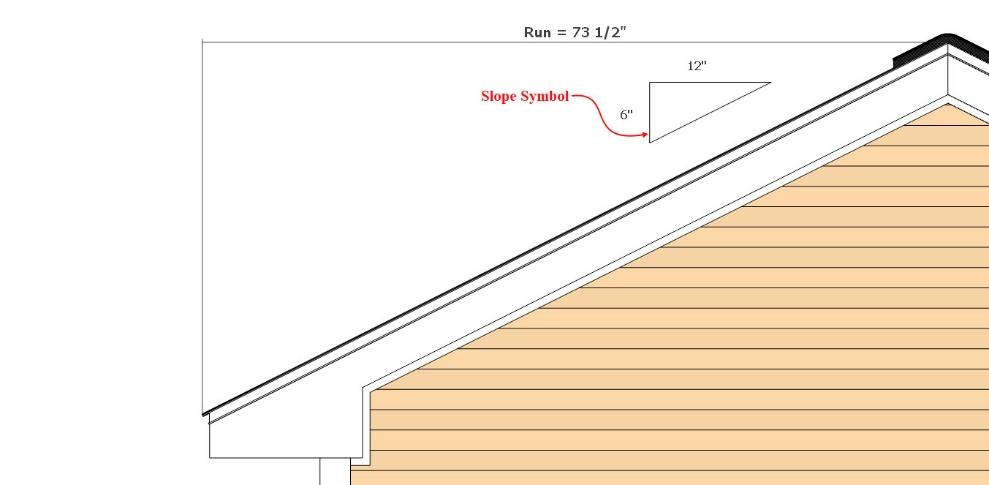Image of a roof with a labeled slope of 6 over 12 and a total run of 73.5 inches

The slope ratio can be used to determine the length of any side of the triangle when the length of one side is also known. For example, the roof shown in Figure 12-21 has a run length of 73-1/2″ the length of the rise can be determined by doing the following:

$$\tfrac{6}{12}"\:\times\:73\:\tfrac{1}{2}"\:=\:36\tfrac{3}{4}"$$

With the length of both the rise and run known, the Pythagorean Theorem can be used to calculate the length of the hypotenuse (slope length) by using the following equation:

$$\sqrt{Rise^2\:+\:Run^2}$$

Or

$$\sqrt{73\tfrac{1}{2}^2\:+\:34\tfrac{3}{4}^2}$$

Or

$$\sqrt{73.5^2\:+\:34.75^2}$$

Or

$$\sqrt{5,402.24\:+\:1,207.56}$$

Or

$$\sqrt{6,609.81}$$

Or

81.3″